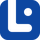# Articles related to "where"

### Subordinating Conjunctions

Subordinating conjunctions joins subordinate or dependent clauses to the main or independent clauses. To know all about these tricky grammatical words, click!

As you know, clauses have subjects and verbs. Adverbial clauses are clauses that function as the adverb of the sentence.

### Wh- Questions

Wh-questions are questions that begin with one of the 'wh-' words. In order to learn about them and to know how to form a wh- question, read this article!

### Where vs. Were vs. Wear

'Where,' 'were,' and 'wear' are three different words but they have similar pronunciations. In this lesson, we will discover more about each of them.

### Where vs. Which

Where and which are wh-words and we have seen the used commonly, but what is their difference? In this lesson, we will discover more about them.

### Conjunctions of Place

The conjunction of place is a kind of subordinating conjunction talking about the whereabouts of something. In this lesson, we will learn all about them.

Relative adverbs are words that give us more description for nouns, the people, places, or things being discussed. Let's get to know them better.

### Wh- Questions

Wh-questions are questions that begin with one of the 'wh-' words. In order to learn about them and to know how to form a wh- question, read this article!

### Where vs. Wherein

'Where' and 'wherein' are close in their appearance; however, they are different. In this lesson, we will find out more about these words.

### Where vs. Whereby

'Where' and 'whereby' are similar in appearance nut are there other similarities? In this lesson, we will learn more about these words.

### Where vs. Whereas

'Where' and 'whereas' are different words but they might confuse learners. In this lesson, we will learn more about these conjunctions.

### Complementizers

Complementizers make a clause into the subject or object of a sentence. In this lesson, we will learn all about them.

### Where

'Where' is a WH-word. In this lesson, we will discuss the rules of using 'Where.'

### When vs. Where

'When' and 'where' are wh-words commonly used in the English language. In this lesson, we will learn more about them and their similarities and differnces.

### Nominal Relative Pronouns

Nominal relative pronouns are also known as free relative pronouns are used to introduce a relative clause. Click here to learn!